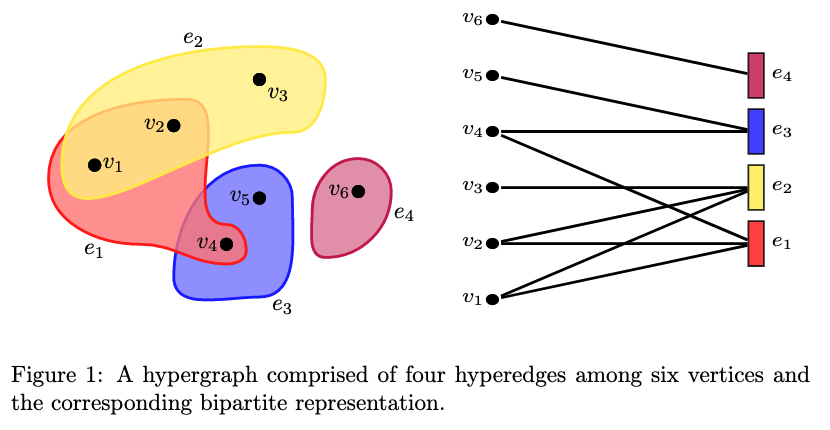# What is a hypergraph?

A graph is a mathematical entity that allows us to represent relationships between pairs objects. The use of graphs is prevalent in genetics, social networks, and paper coauthorship. Taking the last example of paper coauthorship, a graph representation of this would represent individuals on the network as nodes and a pair of nodes will be connected by an edge if the two individuals have coauthored a paper together.

While powerful, the regular graph is restricted to only allowing pairwise interactions to be modelled. In reality, many systems exhibit higher order interaction structures. Using the previous example, it is often the case that a paper is authored by more than two authors. By working together, the collection of authors are greater than the sum of their parts, and there is actually a significant effect present through the interaction of all the authors working together as a group.Hypergraphs allow us to represent these higher-order interactions by replacing the graph’s edge with a hyperedge. A hyperedge is a collection of vertices where it is assumed that all vertices within the hyperedge interact. Using the coauthorship example, a hyperedge would now correspond to a single paper with the all the paper’s authors being part of the hyperedge. We can see this in the above figure. It is perfectly valid for multiple hyperedges to intersect if, for example, a single author collaborates with multiple sets of coauthors on a series of papers.

This atomic essay only scratches the surface of a hypergraph. For more information, the recent paper by Battiston et. al. (2020) is an excellent starting point.